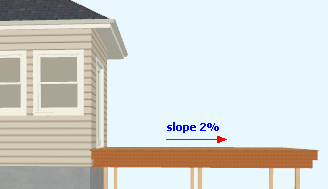Spike's Calculators

# Slope from a Percentage

Quite often you run into something like "minimum slope of deck 2% away from building" or a garage floor requires a minimum slope of 1% or 2%.

This calculator will calculate the drop in inches, the difference in elevation, from a slope expressed as a percentage.##### Example calculation

A deck extending 12 feet 6 inches from the house requires a 2% slope.

Convert feet to inches: 12 * 12 = 144 + 6 = 150 inches
```150 * 2% =
150 * (2 ÷ 100) =
150 * 0.02 = 3"```
The elevation difference in the deck from house to the end of the deck equals 3 inches.

### Slope Percentage Drop

Length ft in
Slope in a Percentage %
Fraction Precision #

#### Results:

 Difference in Height in

#### Calculation

1. length in feet and inches
2. slope in a percentage
3. fraction precision preset @ 16

#### Result

1. slope in inches (drop)